International
Tables for
Crystallography
Volume C
Mathematical, physical and chemical tables
Edited by E. Prince

International Tables for Crystallography (2006). Vol. C, ch. 6.4, pp. 610-611

## Section 6.4.8. The value of x

T. M. Sabinea

aANSTO, Private Mail Bag 1, Menai, NSW 2234, Australia

### 6.4.8. The value of x

| top | pdf |

For the single mosaic block, application of the relationship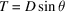leads to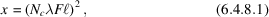whereis the average path length through the block. In the correlated block model, x is also a function of the tilts between blocks and the size of the crystal.

It will be assumed for the discussion that follows in this section that the mosaic blocks are cubes of side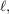and the distribution of tilts will be assumed to be isotropic and Gaussian, given by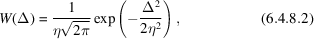where Δ is the angular deviation of the block from the mean orientation of all blocks in the crystal, and η is the standard deviation of the distribution. (The assumption of a Gaussian distribution is not critical to the argument that follows.)

Let the crystal be a cube of side L, and let α be the probability that a ray reflected by the first block is reflected again by a subsequent block. The effective size of the crystal for Bragg scattering of a single incident ray is then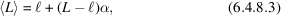while the size of the crystal for all other attenuation processes is L, since, for them, the Bragg condition does not apply. The probability of re-scattering, α, can readily be expressed in terms of crystallographic quantities. The full width at half-maximum intensity of the Darwin reflection curve is given, after conversion to the glancing-angle (θ) scale, by Zachariasen (1945) as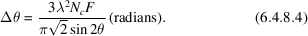The full width at half-maximum (FWHM) of the mosaic-block distribution (6.4.8.2)is derived in the usual way, and the parameter g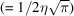is introduced to clear (to 1%) numerical constants. Then α, which is equal to the ratio of the widths, is given by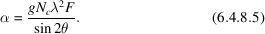Insertion of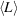[equation (6.4.8.3)] in place ofin equation (6.4.8.1)for x leads to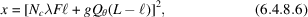where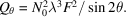### References

Zachariasen, W. H. (1945). Theory of X-ray diffraction in crystals. New York: John Wiley, Dover.